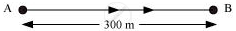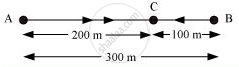Share

# Joseph Jogs from One End a to the Other End B of a Straight 300 M Road in 2 Minutes 30 Seconds and Then Turns Around and Jogs 100 M Back to Point C in Another 1 Minute. - CBSE Class 9 - Science

ConceptMeasuring the Rate of Motion - Speed with Direction

#### Question

Joseph jogs from one end A to the other end B of a straight 300 m road in 2 minutes 30 seconds and then turns around and jogs 100 m back to point C in another 1 minute. What are Joseph’s average speeds and velocities in jogging (a) from A to B and (b) from A to C?

#### Solution

(a) From end A to end BDistance covered by Joseph while jogging from A to B = 300 m

Time taken to cover that distance = 2 min 30 seconds = 150 s

"Average speed"="Total distance covered"/"Total time taken"

Total distance covered = 300 m

Total time taken = 150 s

"Average speed"=300/150=2"m/s"

"Average velocity"="Displacemnet"/"Time interval"

Displacement = shortest distance between A and B = 300 m

Time interval = 150 s

"Average velocity"=300/150=2"m/s"

The average speed and average velocity of Joseph from A to B are the same and equal to 2 m/s.

(b) From end A to end C"Average speed"="Total distance covered"/"Total time taken"

Total distance covered = Distance from A to B + Distance from B to C

= 300 + 100 = 400 m

Total time taken = Time taken to travel from A to B + Time taken to travel from B to C = 150 + 60 = 210 s

"Average speed"=400/210=1.90"m/s"

"Average velocity"="Displacement"/"Time interval"

Displacement from A to C = AC = AB − BC = 300 − 100 = 200 m

Time interval = time taken to travel from A to B + time taken to travel from B to C

= 150 + 60 = 210 s

"Average velocity"=200/210=0.95"m/s"

The average speed of Joseph from A to C is 1.90 m/s and his average velocity is 0.95 m/s.

Is there an error in this question or solution?

#### Video TutorialsVIEW ALL 

Solution Joseph Jogs from One End a to the Other End B of a Straight 300 M Road in 2 Minutes 30 Seconds and Then Turns Around and Jogs 100 M Back to Point C in Another 1 Minute. Concept: Measuring the Rate of Motion - Speed with Direction.
S